# Superconduction is sweetest when served up cold

By
Marta Reina
July 10, 2023
12 minutesIn this article I will pose the basis to understand how superconductivity can be used to built quantum bits. To do so, I will present the Bardeen-Cooper-Schriffer (B.C.S.) microscopic theory, developed in 1957 , that earned the authors a Nobel Prize in 1972. This theory explains the microscopic processes underlying the phenomenon of superconductivity, first observed by Onnes in 1911, while performing experiments on the resistivity of pure mercury. During an investigation conducted around 4 K (-270 °C), the physicist noticed that mercury opposed zero resistance to the passage of electric current . Countless other superconductors were discovered shortly afterwards and, surprisingly, many in the ranks of ambient-temperature metallic bad conductors. All of them showed the same two characteristics:

• a resistivity falling abruptly to zero for temperatures below a material-dependent critical temperature;
• a perfect diamagnetism, i.e. a complete expulsion of interior magnetic field, below this same temperature and a certain temperature-dependent magnetic field (Meissner-Ochsenfeld effect).

Although all superconductors exhibit these behaviours, they have a very different range of temperatures when it comes to showing their magic: so-called type I superconductors have cryogenic critical temperatures while type II have higher ones — up to 120 K — . Also from the magnetic point of view, these two groups are easily distinguishable: the former are characterised by only one critical magnetic field below which a full Meissner-Ochsenfeld effect is showed, while the latter are marked by two critical fields. Below the smallest a perfect diamagnetism is reached, between the two a gradually increasing penetration of the magnetic field lines can be observed, while above the highest any trace of a superconduction and diamagnetism behavior is lost. For our scopes, we are going to focus only on type I superconductors, which includes all the pure metals but Vanadium, Niobium and Technetium.

Right after their discovery, the hype about the subject spread enough that physicists started to wonder about how this phenomenon was practically possible and explainable from a microscopic point of view. This leads us directly to the formulation of the B.C.S. theory. It relies on the intuition that the heroes in this story were the electrons, partially freed from the bad influence of phonons thanks to low temperatures. More precisely, Bardeen originally hypothesised that the superconduction phenomenon was due to a bizarre attractive interaction between electrons, hypothesis later confirmed by Cooper’s theory on the existence of pairs of electrons, generated through a phonon-mediated attractive interaction. Cherry on the cake, Schriffer was able to package the theory by successfully writing a ground state wave function for these couple of electrons.

But… we are not in a rush! Let's start from the very beginning by presenting the main characters of what will soon appear as a wonderful love story.

Let's consider a small part of a conducting solid, containing just a few atoms. These atoms are arranged in a lattice structure and can vibrate due to external causes. Quantumly, such vibrations are described by pseudo-particles: you are finally ready to meet the phonons.

In the same lattice, we have also the opportunity to meet our second main characters: the electrons. The outermost of them in a conductor material are shared among the atoms and, when the sample is subjected to an electric potential difference, an electric field is generated forcing those to move toward the positive pole giving rise to an electric current.

Anyway, it is not so simple. We know that not only is the conductivity limited but also an electric resistance exists. Where do these two features come from?

Standing in between the electrons and their mission is first of all their own profound nature: these particles have a purely quantum property, known as spin. Since it can be mathematically described this way, for the sake of simplicity you can picture it as a spinning of the particle around its own axis, just like the rotation period of a celestial object. Because of this, the electrons along with all the other half-integer spin particles — called Fermions — are subject to Pauli’s exclusion principle: only 2 electrons with opposite spin can be present in a single energy state. The electrons are then forced to gradually stack up to higher energy levels according to a distribution called the “Fermi-Dirac” statistic. The exclusion principle is not limited to electrons in atomic orbitals but to all electrons organised in discrete energy levels, such as conduction energy levels. This means that when the lower conduction energy levels are occupied the energy required to promote valence to conduction electrons is no longer zero and this energy will keep increasing as the population of conduction electrons grows, limiting the conductivity of the material .

Moreover, the interaction between the two classes of roommates is very complicated: the higher the temperature the higher the number of phonons participating to the party. If the crystal lattice was perfect, electron propagation would occur without loss of energy, but that's not the case. Lattice vibrations (phonons) change the rest position of the atoms, and the electrons in their motion notice this and interact with them, losing energy. There is an electron-phonon interaction. Any collision between phonons and electrons counteracts the latter’s motion by negatively affecting the electric current, thus increasing the electrical resistance of the material. Lowering the temperature decreases the number of phonons and, as a consequence, also decreases the value of the electrical resistance to a constant value that will depend only on the defects present.

In this situation, the passage of a conduction electron through the crystal lattice will attract to itself the positively charged ions, causing a movement of the crystal lattice (phonon) and, therefore, an accumulation of positive charge. This distribution of positive charges due to lattice deformation, not being disturbed by thermal agitation, will persist for a time Δt significantly larger compared to the time of electron motion. This is due to ions being much more massive than conduction electrons and, as a consequence, much slower. Therefore, as soon as the first electron is far enough away, and its charge substantially shielded, a second electron will be attracted by the accumulation of positive charge, giving rise to an attractive net interactionbetween the two electrons  (Fig. 1).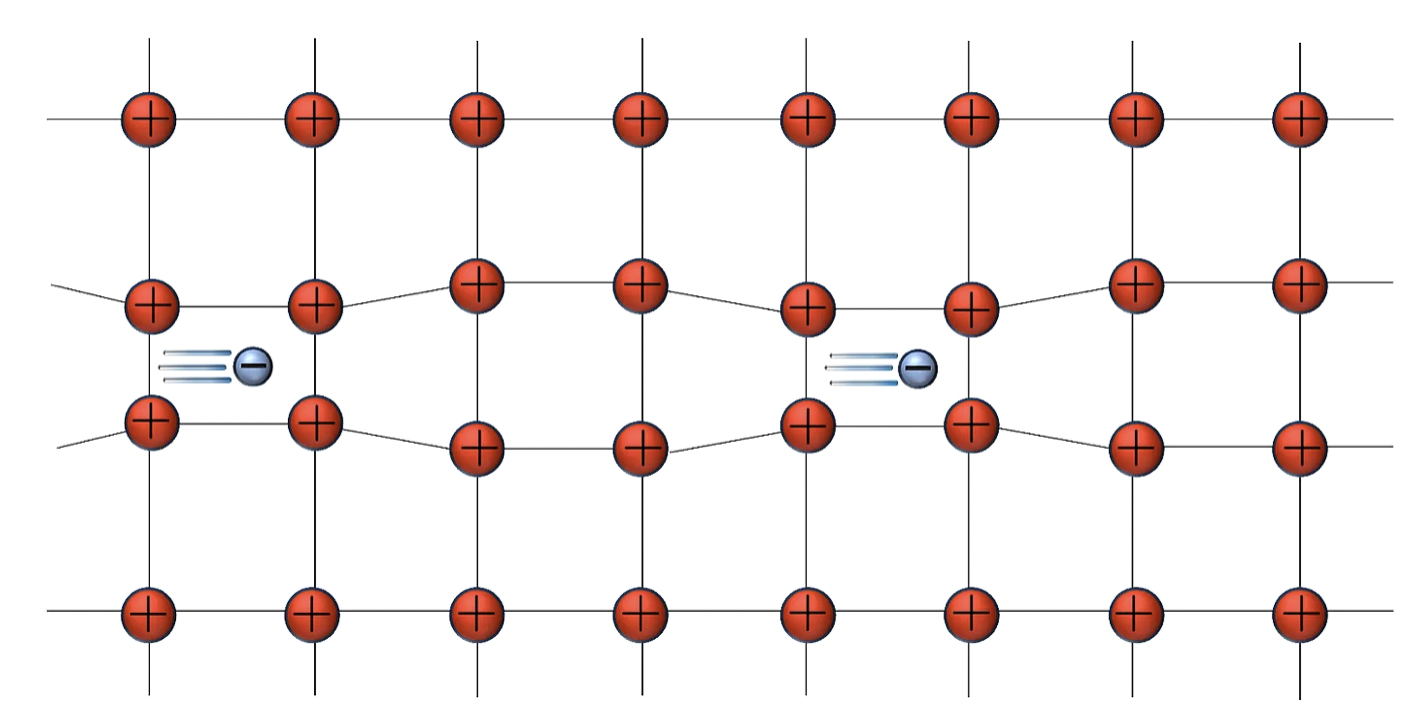Fig. 1 - Schematic representation of how the lattice reacts to the passage of electrons, creating a zone with higher density of positive charge. Due to that, when the first electron is sufficiently shielded, a second electron moves toward that zone.

That's how you can classically explain the creation of this amazing couple, known as Cooper's pair: two electrons are kept together thanks to the interaction of a phonon.

Jokes aside… you won’t be surprised but to dig deep into this story we need to use quantum mechanics and to leave behind our classical intuitive explanation. This is going to help us to understand why, even though low temperature shouldn't solve any Pauli-principle-related issue… they do!

Here we start a more detailed treatment by explaining the interaction underlying the creation of Cooper pairs, called Fröhlich interaction which is schematised by means of Feynmann diagram below (Fig. 2).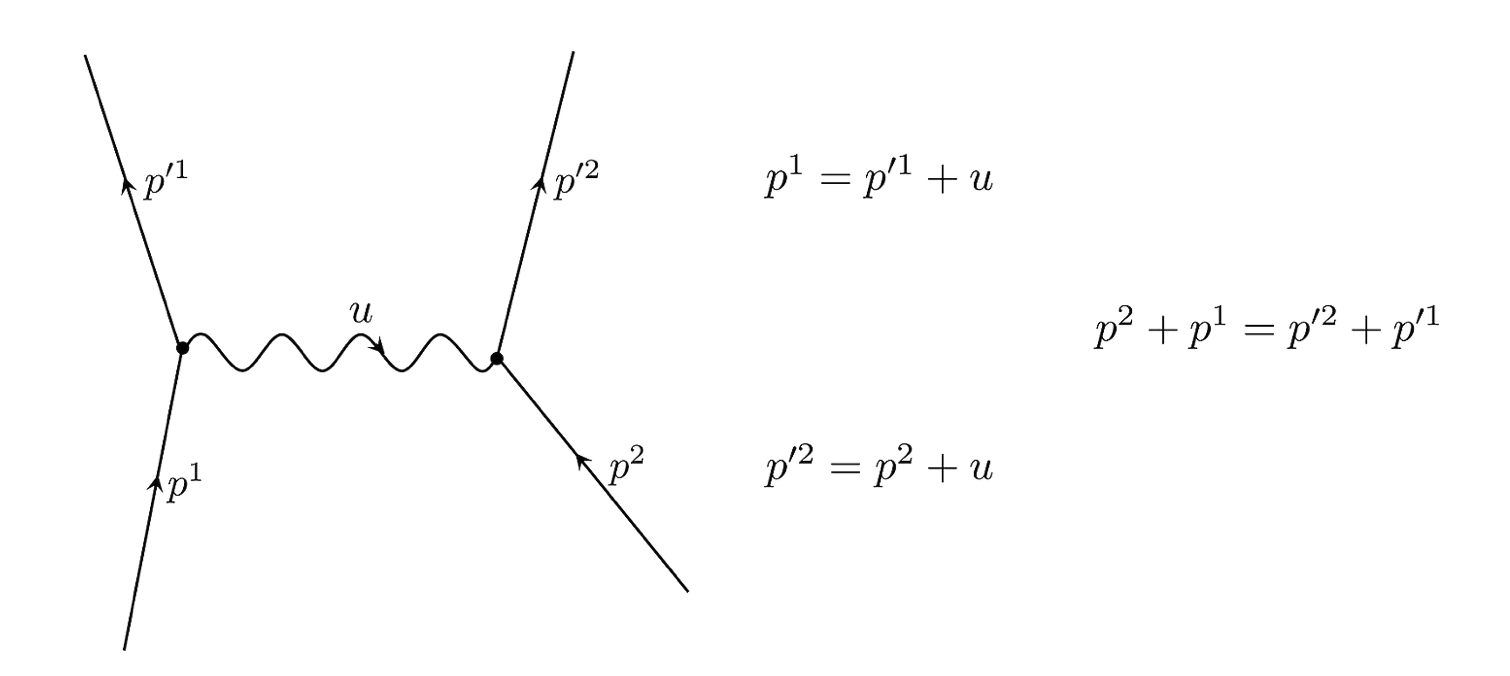Fig. 2 - Representation of the Fröhlich interaction by means of a Feynmann diagram.

Let's consider a conductor at T=0, the scattering of an electron of momentum p¹ with the lattice will excite vibrational modes and, therefore, emit a phonon by losing momentum equal to u = hνᵘ/s, where s is the speed of sound. We therefore have that p¹=p’¹+ u.
The phonon is immediately reabsorbed by an electron with momentum p², having quantity of motion (q.o.m.) equal to p²+u at the end of the process.

In total, clearly, momentum will have been conserved (it could not be otherwise in a collision!) and therefore p¹+p²=p’¹+p’².

Concerning the energy, it is well-known that in quantum mechanics due to the energy-time uncertainty principle, i.e. ΔE ≈ ћ/Δt, a process can happen without instant-by-instant energy conservation. This means that, being the process practically instantaneous, the range of energy values the phonon can take would be extremely wide. Thus, the result is an interaction between the two electrons that exchange momentum (and energy) through the mediation of a — very often virtual — phonon .

I hope I convinced you so far, and I didn't lose any of you in the way, because the hardest part arrives just now: I have to persuade you that an attractive interaction between electron can exist despite the presence of the ineliminable Coulomb repulsion. Just kidding, I hope you are going to take my word for this (but in case you don't, here some nice math for you [4-6]): through considerations and calculations it can be deduced the Fröhlich interaction turns out being a weak attractive interaction between electrons when the energy difference before and after the collision is

It is worth noticing that the few pillars we posed so far are already sufficient to explain why to show a superconductive behavior are those metals being ambient-temperature bad conductors: in order to present superconductive behavior, a good electron-phonon coupling is essential. At ambient temperature, this inevitably causes an high resistivity. Good conductors, such as copper, gold and silver can never exhibit superconductive behavior, even at the lowest temperature.

Come here, bro! It's time we saved some energy!
We know what really drives nature*: energy convenience. So, if such a phenomenon exists, it must be energetically convenient.
Let's once again consider a conductor at T=0: its Fermi-Dirac distribution will take a step shape (Fig. 3), i.e., the probability that states below the Fermi energy are occupied will turn out to be 1, while for electronic states above this same energy will be precisely zero.

In the space of momentum, such a graph can be translated into a sphere of radius pᶠ=(2mᵉϵᶠ)¹ᐟ², called a Fermi sphere. As showed in Fig. 4, all the states on the inside turn out to be occupied, and the electrons occupying them are called the “Fermi sea”, while all the states on the outside turn out to be empty.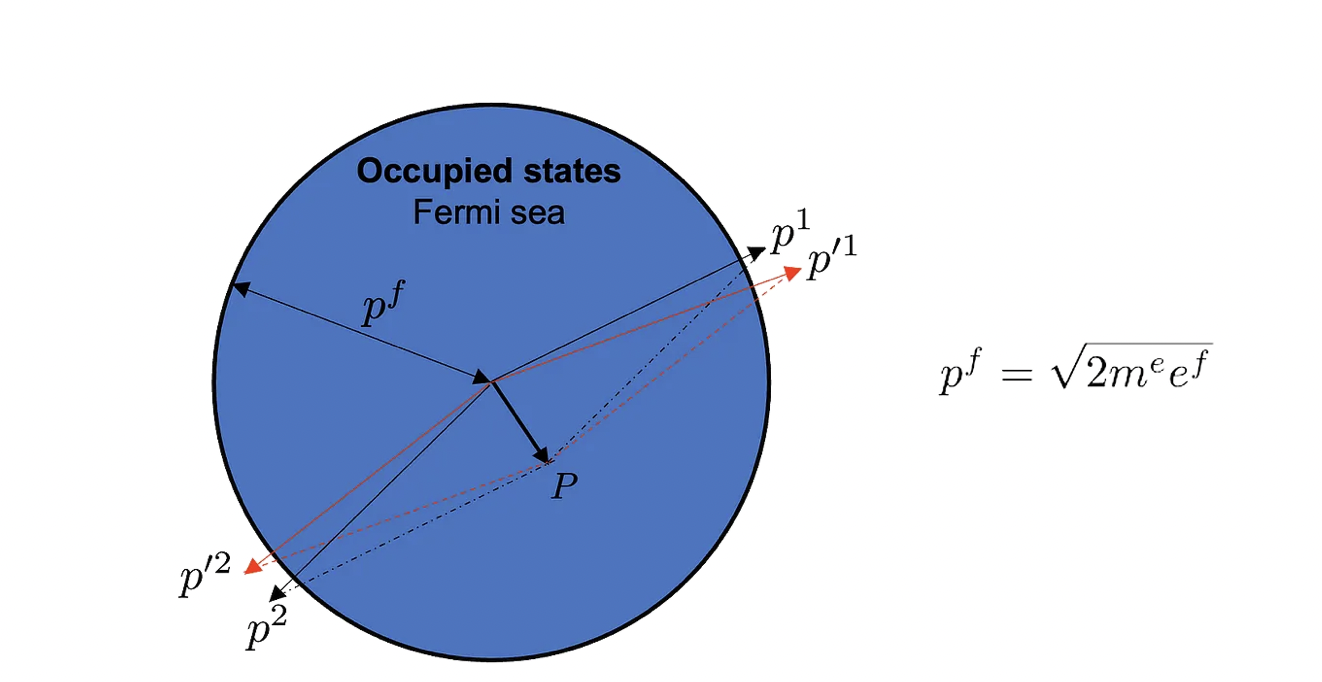Fig. 4 - Fermi sphere of radius pᶠ. All the states within it are occupied. The arrows represent the two electrons we imagined to inject, before and after the collision.

Let us imagine, as Cooper did, to inject two brand new electrons inside our conductor. Since these are subject to Pauli’s exclusion principle, they could only occupy states with p>pᶠ+δp (see Fig. 4).

Let's assume that such electrons are non-interacting, we can then write their wave function as a simple product of wave functions using the Bloch functions ψ, i.e. ϕ(x¹, x², p¹, p²)=ψ(x¹,p¹)ψ(x²,p²). We now want to describe the scattering phenomena that will occur within the lattice: the electrons can still continue to occupy only states with p>pᶠ and, as a result of these scattering episodes, the wave function of the electron pair will be scrambled. The electrons will no longer have well-defined momenta, but they will have a range of available ones. We can write the new wave function as follows

where |aⁱʲ|² represents the probability that the first electron has q.o.m. equal to pⁱ and the second one equal to pʲ. Of course, because of the conservation of the q.o.m. the only non-zero probabilities will be the ones satisfying pⁱ+pʲ=constant=P. The wave function we wrote can be interpreted as a continuous scattering of the two electrons.

As we explained before, they will then be subject to the Fröhlich's interaction and to an attractive interaction corresponds a negative potential energy. However, it can be deduced that to have an appreciable scattering probability ϵ¹-ϵ’¹≈ hνᵘ, and this results in a range for p equal to [pᶠ, pᶠ+mhνᶫ/pᶠ] with νᶫ half the Debye frequency.

Since, however, P must always be conserved, only the ranges of values highlighted in red on the left in Fig. 5 can be assumed by the two electrons.Considering that each scattering event contributes a potential energy -V, it follows immediately that in order to minimize as much as possible the energy of the system the highest number of scattering events has to occur. That is why it is more convenient to form Cooper's pair having opposite q.o.m.: as shown on the right of Fig. 5, the range of allowed q.o.m. for the electrons is maximum when the surface in red — actually a volume — turns out in a complete shell, i.e. for P=0.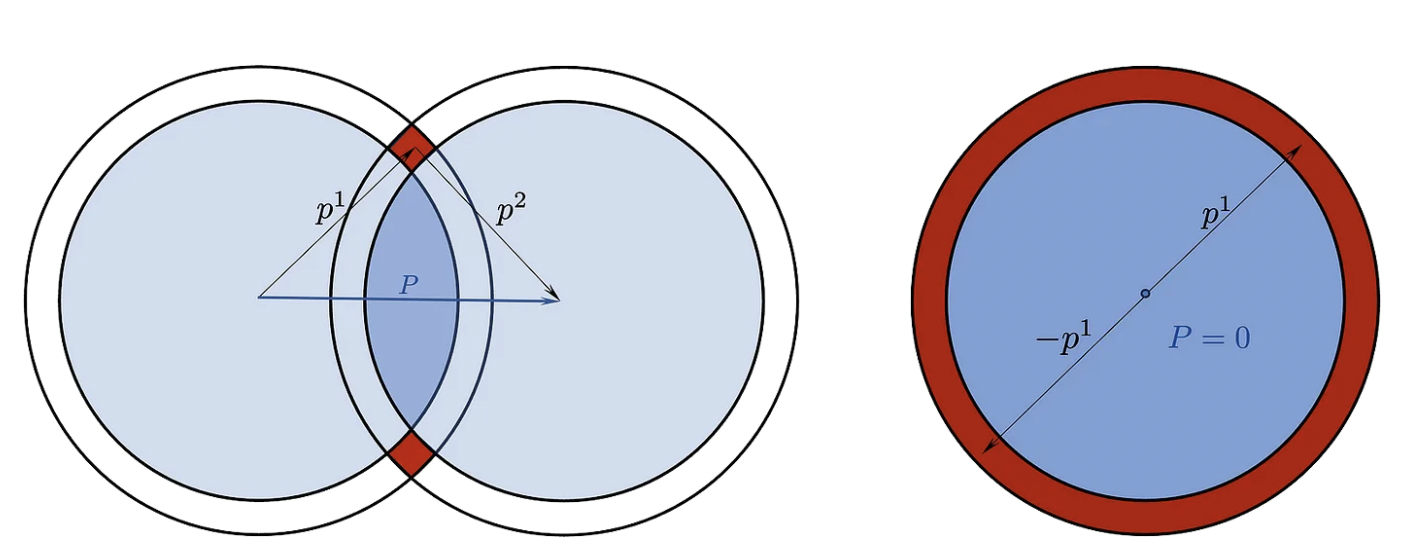Fig. 5 - Left. Volume of available phase space satisfying the conditions of conservation of crystalline momentum for two electrons with p¹ + p² = P. Right. When P=0 and then the two electrons have opposite momentum, the available phase space becomes a complete shell and then results maximized.

On top of that, symmetry arguments make possible to deduce that the value of potential energy V is maximised when the two electrons have opposite spin. Thus, a pair state will be represented by (pⁱ↑, -pⁱ↓).Thanks to these considerations, it is possible to express a pair wave function as

The energy associated with such a pair will be composed by its kinetic energy and the potential energy due to the interaction. Occupying the electrons states in which p>pᶠ, the kinetic energy will be for sure greater than 2ϵᶠ. Nonetheless, precise calculations show that, although W>2ϵᶠ, the amount of negative potential energy is sufficient to obtain a total energy of the pair lower than 2ϵᶠ. Energetically, therefore, two electrons embedded in a metal will prefer to form a bound state and thus a pair, rather than remaining free. The pair formed will be a boson of mass 2mᵉ, charge 2e⁻ and spin 0 or 1.

All for one, and one for all
Cooper’s discovery starts from an unrealistic assumption: not only it considers the interaction between two only electrons, but also it considers the injection of two electrons inside a conductor which we know must exhibit a superconductive state without any manipulation. However, what was discovered also applies very well to the real model. The lowering of energy resulting from the creation of a Cooper pair actually causes the creation of numerous pairs between the rows of electrons having momentum very close to pᶠ. The process of pair creation will end as soon as creating pairs will no more lower the total energy of the system: as soon as too many pairs occupy the allowed states, scattering episodes will be gradually less likely, causing a lowering of the potential energy associated with the pair. There is thus a maximum number of pairs that can be formed. Anyway, what we stated before is true: these pairs cannot be treated as independent objects. All of them need to be treated together, since their wave functions superpose and they still strongly interact due to the Pauli exclusion principle acting on their constituent electrons . Since the conductor is at an extremely low T and the pairs are bosons, they will condense into a single quantum state: a Bose-Einstein condensate. The wave function of such a collective state can be written as follows

At this point, by a process of minimisation of the total energy of the system under probabilistic constraints, it is possible to find an expression for the probability |hᵖ|² that a pair state is occupied or not. The value of this probablity as a function of the energy ϵᵖ=ϵⁱ-ϵᶠ is plotted in Fig.6.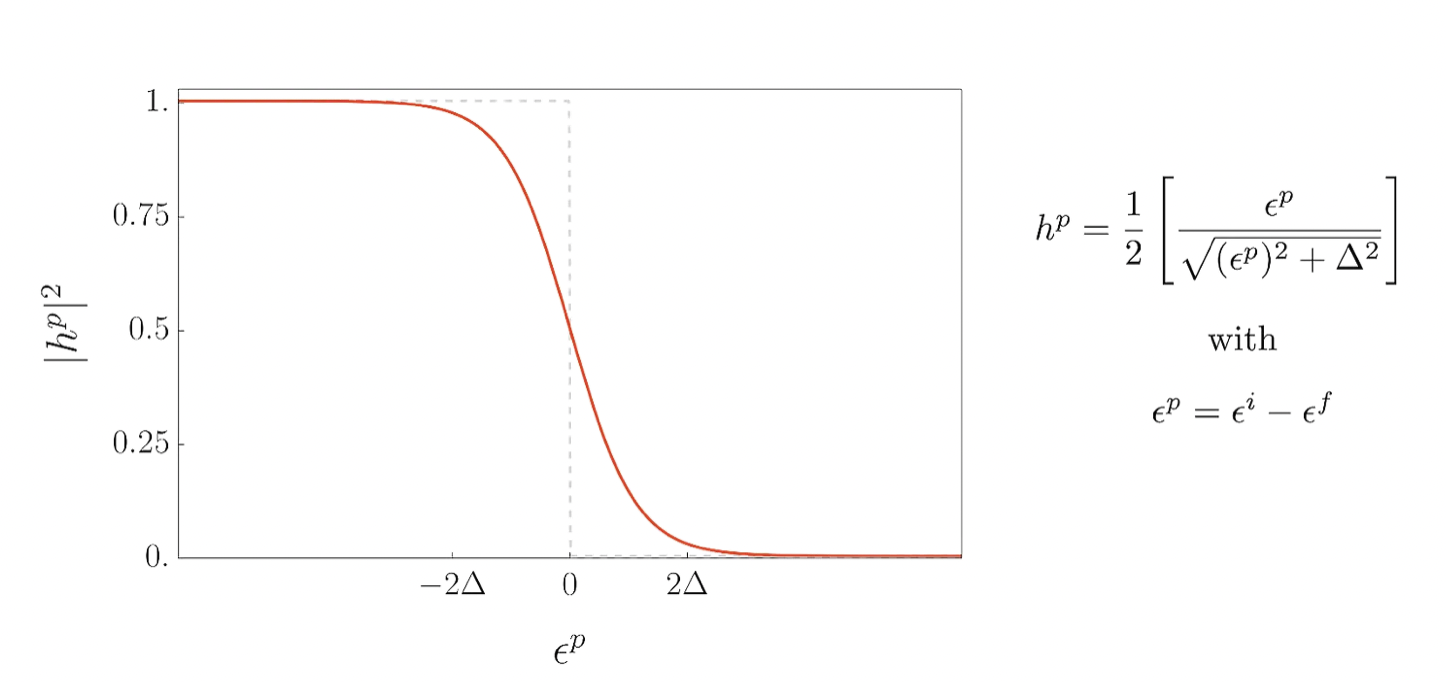Fig. 6 - Probability for a couple state to be occupied at T=0 compared to the Fermi-Dirac distribution shape at the same temperature.

In the ground state B.C.S., in fact, the distribution function of the couples does not exhibit a discontinuity even at T=0, as is the case with the Fermi-Dirac distribution. Looking again at the expression of hᵖ, it is worth mentioning the quantity Δ, having the dimensions of an energy: this parameter turns out to be of extreme importance since it represents half of the minimum amount of energy that must be supplied to break the bound between the two electrons, i.e. to turn a Cooper pair in two single free electrons. In other words, 2Δ represents the energy gap between the superconducting state and the normal state [This may bring to your mind semi-conductors]. Including in Δ its natural dependance on the temperature, we are able to explain why superconductive behaviors occur only below a certain critical temperature: the energy gap after a linear trend up to 0.6 Tᶜ falls to zero around the critical value. Above Tᶜ the formation of Cooper pairs is no longer possible: there is no longer an energy gap.

But this is only part of the story I want to tell you…
It is time to discover how all this can be used to boost our technologies. What goes
very well with very loooooow temperatures? Yeah, qubits, you are right! Have you ever heard about superconductive qubits? If you want to know more, don't lose my next medium article!

At ColibrITD, we believe that understanding deeply how each technology works is essential for a solid background in order exploit at best each hardware. Mastering these concepts, including how a superconductive qubit can be built, is the key to starting the quantum computing revolution.

*Okay, okay! You are right! To be fair it is better to speak about free energy, but this changes nothing for our scopes.

References:
 J. Bardeen, L.N. Cooper, and J.R. Schrieffer, Theory of Superconductivity, Phys. Rev. 108, 1175 (1957).
 H.K. Onnes, The Resistance of Pure Mercury at Helium Temperatures, Commun. Phys. Lab. Univ. Leiden, 12, 1 (1911).

 S. Fujita, K. Ito and S. Godoy, Quantum Theory of Conducting Matter — Superconductivity. Springer, New York (2009).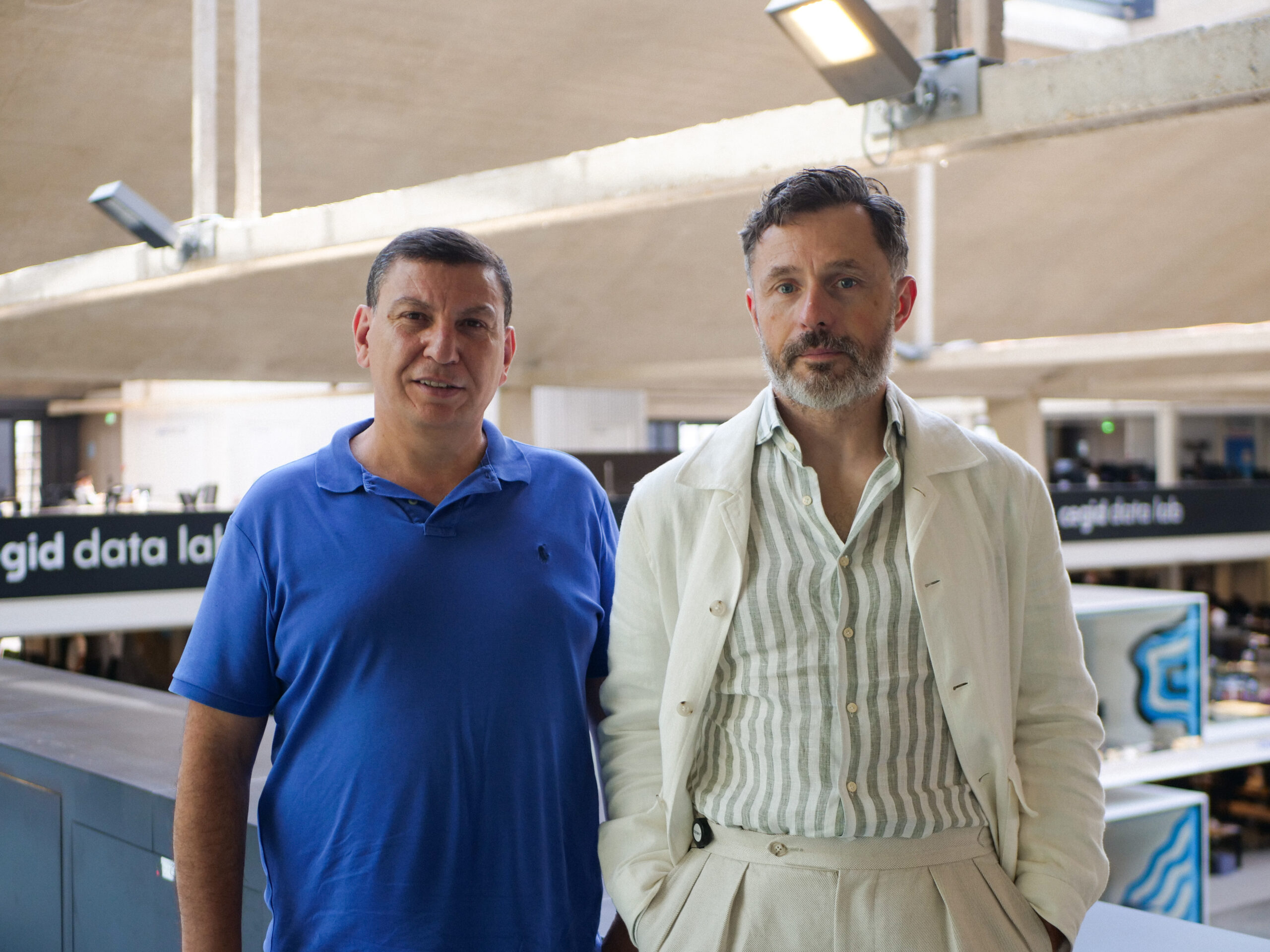Marta Reina